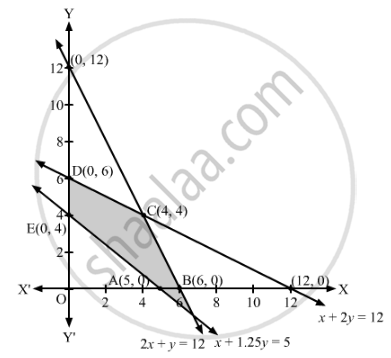# A Manufacturer Has Three Machine I, Ii, Iii Installed in His Factory. Machines I and Ii Are Capable of Being Operated for at Most 12 Hours Whereas Machine Iii Must Be Operated Atleast 5 Hours a Day. - Mathematics

Sum

A manufacturer has three machine I, II, III installed in his factory. Machines I and II are capable of being operated for at most 12 hours whereas machine III must be operated for atleast 5 hours a day. She produces only two items M and N each requiring the use of all the three machines.
The number of hours required for producing 1 unit each of M and N on the three machines are given in the following table:

 Items Number of hours required on machines I II III M 1 2 1 N 2 1 1.25

She makes a profit of ₹600 and ₹400 on items M and N respectively. How many of each item should she produce so as to maximise her profit assuming that she can sell all the items that she produced? What will be the maximum profit?

#### Solution

Suppose x units of item M and y units of item N are produced to maximise the profit.

Since each unit of item M require 1 hours on machine I and each unit of item N require 2 hours on machine I, therefore, the total hours required for producing x units of item M and y units of item N on machine I are (2x + y). But, machines I is capable of being operated for at most 12 hours.

∴ 2x + ≤ 12

Similarly, each unit of item M require 2 hours on machine II and each unit of item N require 1 hour on machine II, therefore, the total hours required for producing x units of item M and y units of item N on machine II are (x + 2y). But, machines II is capable of being operated for at most 12 hours.

∴ x + 2≤ 12

Also, each unit of item M require 1 hour on machine III and each unit of item N require 1.25 hour on machine III, therefore, the total hours required for producing xunits of item M and y units of item N on machine III are (x + 1.25y). But, machines III must be operated for atleast 5 hours.

∴ x + 1.25≥ 5

The profit from each unit of item M is ₹600 and each unit of item N is ₹400. Therefore, the total profit from x units of item M and y units of item N is ₹(600+ 400y).

Thus, the given linear programming problem is

Maximise Z = 600+ 400y

subject to the constraints

2x + ≤ 12

x + 2≤ 12

x + 1.25≥ 5

x, y ≥ 0

The feasible region determined by the given constraints can be diagrammatically represented as,The coordinates of the corner points of the feasible region are A(5, 0), B(6, 0), C(4, 4), D(0, 6) and E(0, 4).

The value of the objective function at these points are given in the following table.

 Corner Point Z = 600x + 400y (5, 0) 600 × 5 + 400 × 0 = 3000 (6, 0) 600 × 6 + 400 × 0 = 3600 (4, 4) 600 × 4 + 400 × 4 = 4000    → Maximum (0, 6) 600 × 0 + 400 × 6 = 2400 (0, 4) 600 × 0 + 400 × 4 = 1600

The maximum value of Z is 4000 at x = 4, y = 4.

Hence, 4 units of item M and 4 units of item N should be produced to maximise the profit. The maximum profit of the manufacturer is ₹4,000.

Concept: Graphical Method of Solving Linear Programming Problems
Is there an error in this question or solution?

#### APPEARS IN

RD Sharma Class 12 Maths
Chapter 30 Linear programming
Exercise 30.4 | Q 48 | Page 56